# Metasurface Antenna Modeling

Metasurface antenna is a new concept in surface antennas, which take the advantage of periodic boundary of each radiation unit cells to group radiation unit in a more compact space. This example presents the design, modeling and analysis of metasurface antenna in Antenna Toolbox. We present the work in .

We discuss the construction of unit radiation element with Antenna Toolbox objects and functions. And we use infiniteArray object and rectangularArray object to construct the large antenna array, on which we perform pattern and current analysis at the designed frequency.

The unit radiator presented in  is a cross I beam structure, with optimized probe feed in one arm. The unit cell has a dimension of 3cm, and gap of 0.5mm. Detailed geometry information is shown below.

```length = 14.75*1e-3; width = 3*1e-3; s = 0.25*1e-3; thickness = 1.5*1e-3; viaDia = 0.5*1e-3*2; feedWidth = viaDia/2; gndLength = length+2*s; gndWidth = gndLength;```

We use the customAntennaGeometry object to construct the top cross I beam radiator, and place it into a reflector to form the unit radiator structure. In this simplified geometry, we remove the substrate from the design, which increases the radiation frequency to 9.14GHz, higher than presented in .

```s1 = antenna.Rectangle('Length',length,'Width',width); s2 = antenna.Rectangle('Length',width,'Width',length); s3 = antenna.Rectangle('Length',feedWidth,'Width',feedWidth); [~]=translate(s3,[-5*1e-3+feedWidth/2 0 0]); pr1 = getShapeVertices(s1); pr2 = getShapeVertices(s2); pr3 = getShapeVertices(s3); radiator = customAntennaGeometry('Boundary',{pr1,pr2,pr3},'Operation','P1+P2+P3'); radiator.FeedLocation = [-5*1e-3 0 0]; radiator.FeedWidth = feedWidth; ant = reflector('Exciter',radiator,'GroundPlaneLength',gndLength,'GroundPlaneWidth',gndWidth,... 'Spacing',thickness,'EnableProbeFeed',true); figure; show(ant);```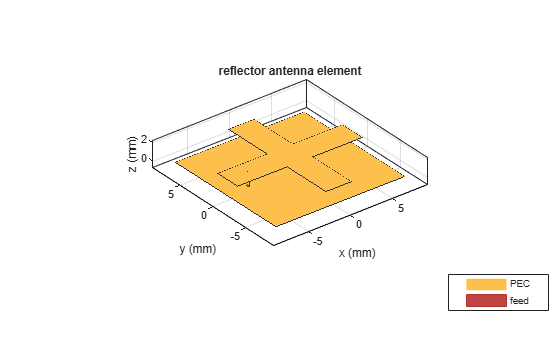### Mesh and Analyze the unit structure.

We manually mesh to control the mesh size generated in analysis.

```figure; mesh(ant,'MaxEdgeLength',0.1);```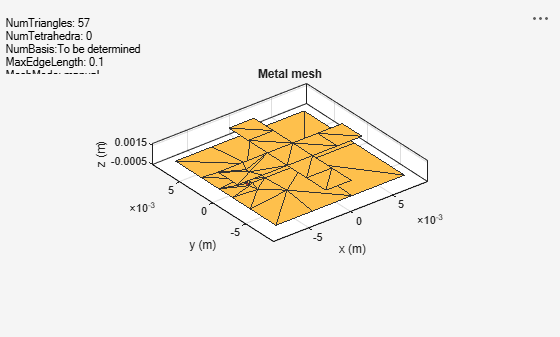```freq = 9.14e9; figure; impedance(ant,freq*[0.8:0.01:1.1]);```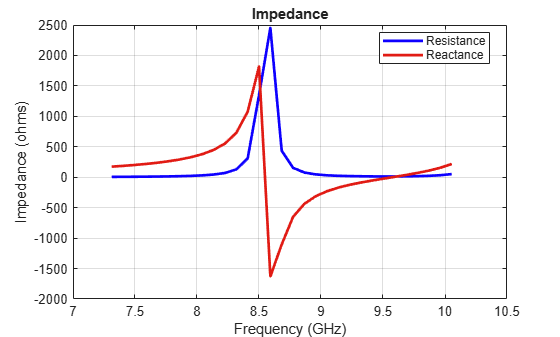### Implement the periodic boundary and analyze

In order to include the periodic boundary effect on the unit radiator, we take the designed unit radiator as the element in infiniteArray object in antenna toolbox, which constructs an infinite antenna array with the designed radiator. Numerically, it utilizes a special green's function in MOM algorithm. The coupling effect between adjacent unit radiator are modeled.

```infArray = infiniteArray('Element',ant); figure; show(infArray);```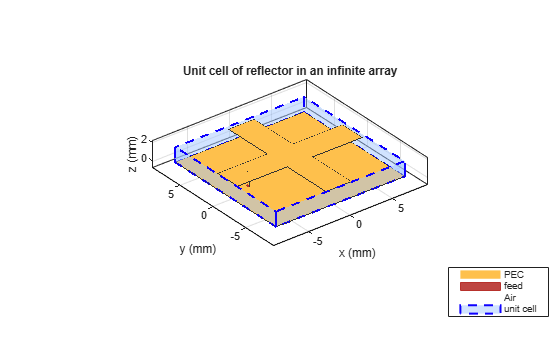```figure; current(infArray, freq);```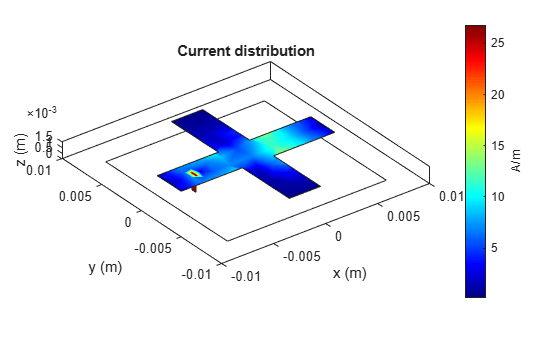### Finite array analyze

In order analyze the coupling effect on a finite size array, we construct a 5 by 5 element rectangularArray with the designed radiator.

```array = rectangularArray('Element',ant); array.Size = [5 5]; array.RowSpacing = gndLength; array.ColumnSpacing = gndWidth; figure; show(array);```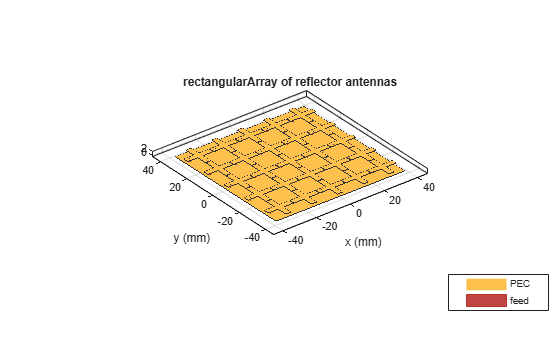The pattern and current analysis on the full array are shown below.

```figure; pattern(array,freq);```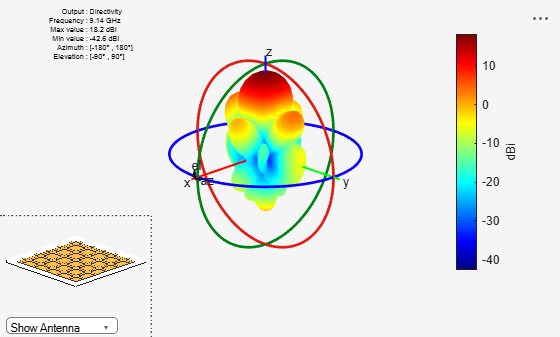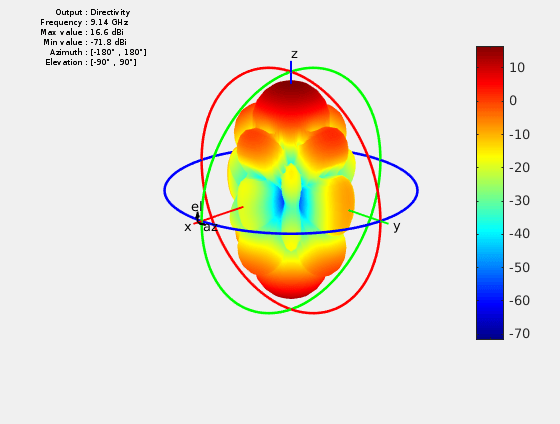```figure; current(array,freq);```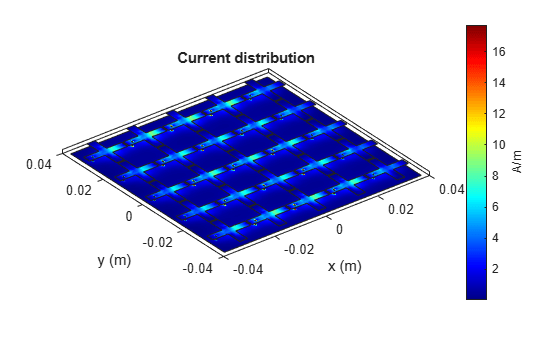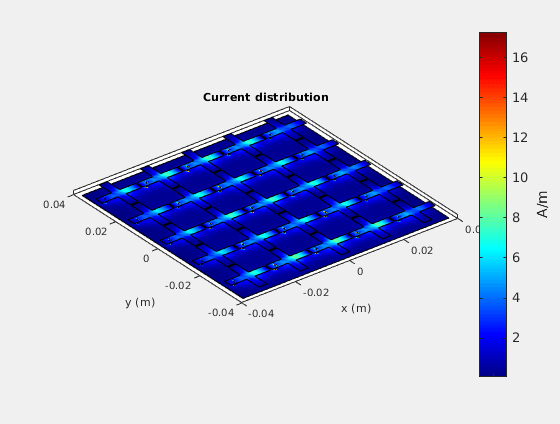### Conclusion

The current and pattern modeling result obtained from the finite array matched similar result reported in .

### References

 M. E. Badawe, T. S. Almoneef, O. M. Ramahi, 'A True Metasurface Antenna', Nature, 6,19268, 2016